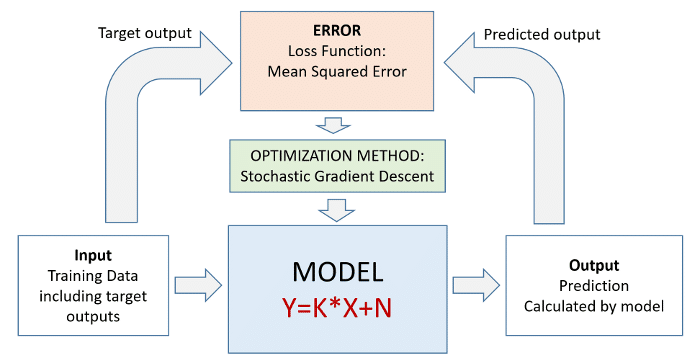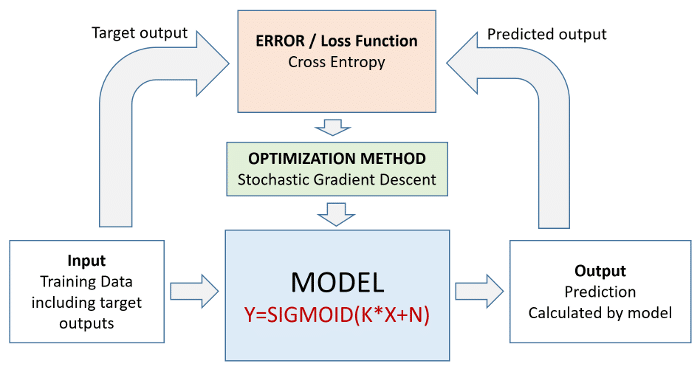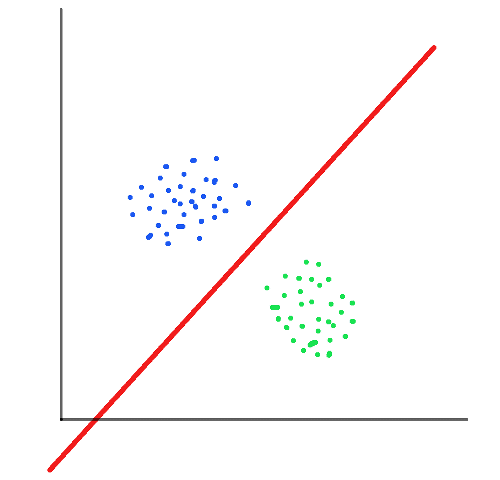# From Basic Machine Learning to Deep Learning in 5 Minutes

Deep learning algorithms evolved from well known mathematical procedures, and the idea of this post is to give a brief overview of that evolution.
That will give you a big picture and better understanding  how they work in general and what are they used for, without going into heavy math.
If you are not familiar with machine learning at all, we recommend you to read our Machine Learning Tutorial for Java Developers first.

## Supervised Learning – Learning From Mistakes

The basic principle used by a number of machine and deep learning  algorithms is called supervised learning. You can think of it as learning from mistakes, since it uses iterative procedure to find a minimum of PREDICTION ERROR of a machine learning model for the given training data. The error is usually expressed as a difference between output predicted by the model and actual/target output which is given as a part of training data.  This error is calculated using so called LOSS FUNCTION. Mathematical procedure for finding minimum of the error/loss function is known as OPTIMIZATION METHOD.  Following this general outline as shown in picture bellow, different machine learning algorithms can be implemented using different models, loss functions and optimization methods. This includes linear regression, logistic regression, feed forward and convolutional neural networks which are explained below.Key Concepts: Error, Loss Function, Optimization
Deep Netts API: LossFunction, LossType, OptimizerType

## Linear Regression

Linear regression is a very basic type of supervised machine learning, which tries to find the best possible straight line to describe main trend in underlying training data. Starting from some random position, it adjusts/moves the line until it finds the position that gives the minimum total average distance of data points from the line. It uses MEAN SQUARED ERROR (MSE) as a loss function and STOCHASTIC GRADIENT DESCENT (SGD) as an optimization algorithm. Stochastic gradient descent optimization method knows how to move line into direction which will likely lower the error. In its basic form, for one input x, and one output y, the model of this algorithm is basic mathematical formula for straight line y=k*x+n and training comes down to figuring out parameters k and n. The same procedure can be applied to problems with multiple inputs.Linear Regression as Supervised Learning AlgorithmLinear Regression finds best straight line through a set of given input points

 Key Concepts: Mean Squared Error, Stochastic Gradient Descent Deep Netts API: LossType.MEAN_SQUARED_ERROR, OptimizerType.SGD Java Code Example: LinearRegression.java

## Logistic Regression

Logistic regression is a basic binary (yes/no) classification algorithm, that works in a same way as linear regression, just instead of adjusting straight line, it adjusts so called SIGMOID function. The output of a logistic regression is probability that given input belongs to some category (eg. spam/not spam, fraud/not fraud). Although Mean Squared Error can be used as a loss function, good practice is to use another type of loss functions called CROSS ENTROPY, which are better suited for classification problems. The basic principle is same: it uses optimization method to find values for parameters of  sigmoid function that give the minimum of the loss function (prediction error for the given data set).Logistic Regression as a Supervised LearningLogistic Regression performs simple separation of two groups of input points(also known as linear classification)

 Key Concepts: Sigmoid, Cross Entropy Loss Deep Netts API: ActivationType.SIGMOID, LossType.CROSS_ENTROPY Java Code Example: Logistic Regression.java

## Feed Forward Neural Network

Feed Forward Neural Network is a directed graph in which each node performs logistic or linear regression. It can be used to solve classification and regression problems, that is to predict a category or numeric value, for the given input.Architecture, components and operation modes of a Feed Forward Network

The nodes are grouped together in a ordered sequence of so called LAYERS, and each node in layer is connected to all nodes in the next layer. Each node performs calculation which takes input from all nodes from previous layer.  It has two modes of operation: training and prediction mode.Feed Forward Network as Supervised Learning

All these nodes can be trained together using BACKPROPAGATION algorithm, which is an extension of the same learning algorithm used in Linear and Logistic regression. Backpropagation algorithm performs error minimization procedure on all nodes in the network, and it propagates error from output across all layers. Network accepts external input at INPUT LAYER which distributes that input onto next (hidden) layer. Every hidden layer performs transformation of its inputs and sends it forward to the next layer. The last layer in a sequence is OUTPUT LAYER. The transformation performed in layers is called ACTIVATION FUNCTION, and beside linear and logistic there are few more that are commonly used in neural networks (like Tanh and ReLU).
Feed forward neural networks can be used for classification and regression problems depending of chosen activation and loss function types.
It has two modes of operation learning (or training mode) in which network adjusts internal parameters in order to minimize prediction error, and  prediction mode in which trained network attempts to predict output for the given input.Non-trivial Classification using Feed Forward Network

 Key Concepts: Feed Forward Neural Network, Layer, Backpropagation, Activation Function Deep Netts API: FeedForwardNetwork, BackpropagationTrainer, ActivationFunction, ActivationType, InputLayer, OutputLayer, FullyConnectedLayer Java Code Example: IrisFlowersClassification.java

## Convolutional Neural Network

Convolutional neural networks are neural networks specialized for image recognition tasks.  You can think of them as a Feed Forward Networks with trainable image filters in front. These image filters perform photoshop-like image filtering (in so called convolutional layers) and resizing (in pooling layers). These image filters (also known as kernels) are able to learn to extract, and recognize  patterns from input image.
Convolutional networks can be used for visual recognition tasks like image classification and object detection. They are trained also with Backpropagation algorithm, same as Feed Forward Networks.Architecture of the Convolutional Neural NetworkImage ClassificationSupervised Learning of Convolutional Network

 Key Concepts: Convolution filters, Convolutional neural network, image classification Deep Netts API: ConvolutionalNetwork, ConvolutionalLayer, MaxPoolingLayer Java Code Example: MnistHandwrittenDigitRecognition.java Handwritten digit classification

#### FREE TRIAL OF FULL FEATURED DEEP LEARNING IDE

Sign up for Deep Netts Platform Free Trial to learn how it can help you to get into deep learning even faster and easier.Scroll to Top